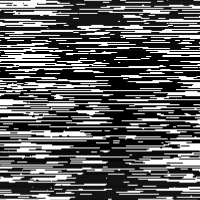# Exchange rows transform

Hi everyone,

We are working on a segmentation model to localize a position in images similar to this below (and corresponding mask):We would like to apply a custom transform to randomly exchange rows (not columns!) in the image. We are having trouble finding a transform function that can do this in v2. In v1 there was Jitter which could randomly exchange pixels across all axes. Is there a version of this transform in v2 that has an option to exchange entire rows? If not, does anyone have ideas for a custom transform we could write to accomplish random row exchange?

Thank you!

1 Like

Watching to see any answers here! I’ve had a hard time figuring out how to do anything with jitter in v2.

1 Like
``````def permute_rows(img, k=5):
"permute k rows on Tensor"
n = img.shape[-2]
rows_idxs = L(random.choices(range_of(n), k=k))
idxs = rows_idxs.sorted()
img[..., idxs, :] = img[..., rows_idxs, :]  #batch compatible
return img
``````

and then as a Transform:

``````class RandPermute(InplaceTransform):
"Permute rows on image, all batch identically"
split_idx,order=0,98 #only apply on train set, before Normalize
def __init__(self, k=5):
self.k = k

def encodes(self, img: TensorImage):
return permute_rows(img, k=self.k)
``````
2 Likes
1 Like

Thank you, @tcapelle ! This is exactly what I was looking for!!